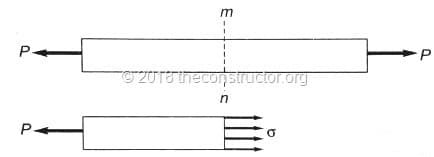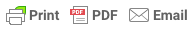Sorry, you do not have permission to ask a question, You must login to ask question. Become VIP Member

Stress is resistance provided by structural material against deformation. Stress is measured in terms of load per unit area and is expressed in MPa or N/mm2.

## Types of Stress in Structural Material

Stress is classified based on its nature and are of following types:

1. Normal Stress
2. Shear Stress

### 1. Normal Stress

Normal stress in a structural material is always perpendicular or normal to the cross-section at any section. It is represented by ? (sigma). Normal stresses are of two types, namely:

#### 1. Axial Stress or Direct Stress

Axial stress is produced when the axial force acts at the center of gravity of the cross-section of structural material. For any Prismatic body with axial loading, axial or direct stresses are uniform across the cross-section.

Axial stresses can be tensile stress or compressive stress. Tensile stresses are considered as positive stresses and compressive stresses as negative stress while computing stress and strains.#### 2. Bending Stress

Bending stresses in a structural material is produced by bending moment experienced by it. This stresses in maximum at the extreme fiber from the neutral axis and is zero at the neutral axis.

Bending stresses are also tensile and compressive. Tensile bending stresses are considered as positive and compressive bending stresses are considered negative for calculations.& Ad Free!

GET VIP MEMBERSHIP NOW!### 2. Shear Stresses or Tangential Stresses

Shear Stresses are resistance provided by the material against shearing force. Shear stress is calculated by dividing the shear forces in the plane of cross-section by corresponding area. It is also expressed as MPa or N/mm2.

Shear Stresses can be classified as direct shear stress and torsional shear stress.

1. Direct shear stress – It is produced in the structural material by the action of direct shear force on the surface.

2. Torsional shear stress – This stress produced in the structural material when the member is subjected to torsional moment or torque.## Sign Conventions of Shear Stresses

For the calculation of various types of stresses in a structural member, a uniform sign convention is used to avoid confusion and errors while computing. Following sign convention can be used for your calculation: